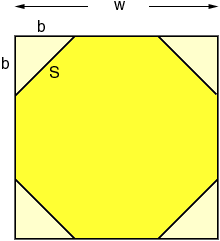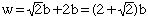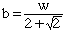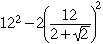What is the actual square footage of a 12' octagon? Hi, I am going to assume that you have a regular octagon and that the width is 12 feet (w in the diagram below). I am going to think of this octagon as a square with the four corners cut off as in the diagram.The four pieces cut off can be arranged into two squares with sides of length b feet and hence the area of the octagon is w 2 - 2 b 2 square feet. Since w = 12 feet, all that is needed is to find b. From the diagram w = b + S + b = S + 2b. Also each of the cut off pieces is a right triangle and hence, by Pythagoras' Theorem, S 2 = b 2 + b 2 = 2 b 2 ThusorHence the area issquare feet which is approximately 119 square feet. Cheers, Penny Go to Math Central